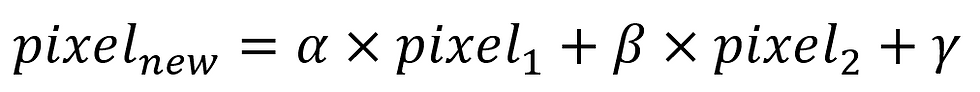top of page

# Start Python for Image Processing with OpenCV and ML　PART 2Continuing from our previous part, to get a better understanding of how computers treat digital images, let me introduce a bit more about grayscale images and RGB images.

Grayscale images are typically represented as two dimensional arrays, where each element in the array represents the intensity value of a single pixel in the image. The dimensions of the array correspond to the height and width of the image, and each element in the array represents the intensity value of the corresponding pixel in the image. The intensity value is usually 8 bit value ranging from 0 to 255, where 0 represents black and 255 represents white.

For example, if you have a grayscale image with dimensions of 3x3 pixels, you could represent it as a two-dimensional array with dimensions of 3x3. Each element in the array would represent the intensity value of the corresponding pixel in the image as follows.Here is how you can create grayscale images as a two-dimensional array using numpy.As you can see, it is amazing that handling grayscale image is literally handling a two-dimensional array, isn’t it?

Now, as for RGB images, a third dimension is added to represent the color channels. In grayscale images, each pixel is represented by a single intensity value ranging from 0-255. In contrast, RGB images have three color channels: red, green, and blue. Each pixel in an RGB image is represented by three intensity values, also ranging from 0 to 255.The combination of these 3 color channels creates a full-color image.Here is how you can create RGB images as a three-dimensional array using numpy.To convert an RGB image to grayscale, a common method is to take the weighted average of the red, green, and blue color channels at each pixel location, and assign the resulting value as the grayscale intensity value for that pixel. This effectively collapses the 3 color channels into a single grayscale channel. Another method is to choose one of the color channels and use its intensity values as the grayscale intensity values for the entire image. For example, the green channel is often chosen because it provides the best balance of brightness and contrast for most images.

OpenCV's cvtColor method applies the following formula to convert an RGB image to grayscale:

Y = 0.299 R + 0.587 G + 0.114 B

where R, G, and B are the red, green, and blue color channels of the RGB image, and Y is the resulting grayscale intensity value. The green channel is given the largest weight because the human eye is most sensitive to green light, while the red and blue channels are given smaller weights. In the previous part, we introduced how to split RGB images into single channel color image by cv2.split method and how to transfer an RGB image to grayscale image using cv2.cvtColor so we won’t repeat them here.

To extend a grayscale image to an RGB image, the grayscale intensity value for each pixel is copied into all 3 color channels, resulting in a grayscale image with three identical channels. This image can then be modified or transformed using various techniques designed for RGB images.

As you may noticed in the code examples above, we retrieved and changed some certain pixel color. If we want to change the color of some certain area, we will use what we called ROI, which is the Region of Interest.We will introduce more applications in the upcoming tutorials.

When we have two images in the same shape, another thing we could do is to blend the two images. Usually, we set gamma 0 in most cases.